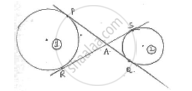Share

# In Fig Common Tangents Pq and Rs to Two Circles Intersect at A. Prove that Pq = Rs. - CBSE Class 10 - Mathematics

ConceptCircles Examples and Solutions

#### Question

In fig common tangents PQ and RS to two circles intersect at A. Prove that PQ = RS.

#### Solution

ConsiderTwo circles namely (i) & (ii) as shown with common tangents as PQ and RS.

We know that

The tangents from external point to the circle are equal in length.

From A to circle (i) AP = AR … (i)

From A to circle (ii), AQ = AS …. (ii)

(i) + (ii) ⇒ AP + AQ = AR + RS

⇒ PQ = RS

Is there an error in this question or solution?

#### Video TutorialsVIEW ALL 

Solution In Fig Common Tangents Pq and Rs to Two Circles Intersect at A. Prove that Pq = Rs. Concept: Circles Examples and Solutions.
S